# How to Rotate an Image with JavaScript

## 1. Rotate the image counter-clockwise, 2. Customize image transform origin, 3. Rotate image on button click, 4. Rotate image incrementally.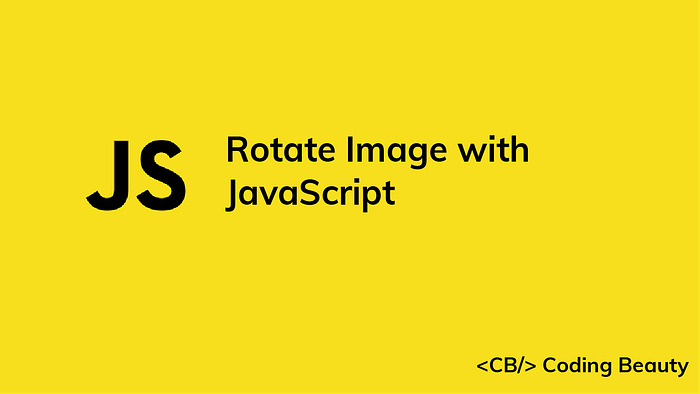To rotate an image with JavaScript, access the image element with a method like `getElementById()`, then set the `style.transform` property to a string in the format `rotate({value}deg)`, where `{value}` is the clockwise angle of rotation in degrees.

Consider this sample HTML:

index.html

``````<!DOCTYPE html>
<html>
<title>JavaScript Rotate Image</title>
<body>
<div style="margin: 8px">
<img src="my-image.jpg" width="300" />
<!-- The image element to rotate -->
<img id="rotated" src="my-image.jpg" width="300" />
</div> <script src="index.js"></script>
</body>
</html>
``````

Here's how to easily rotate the `#rotated` image element from JavaScript:

index.js

``````// Access DOM element object
const rotated = document.getElementById('rotated');
// Rotate element by 90 degrees clockwise
rotated.style.transform = 'rotate(90deg)';
``````

And this will be the result on the web page: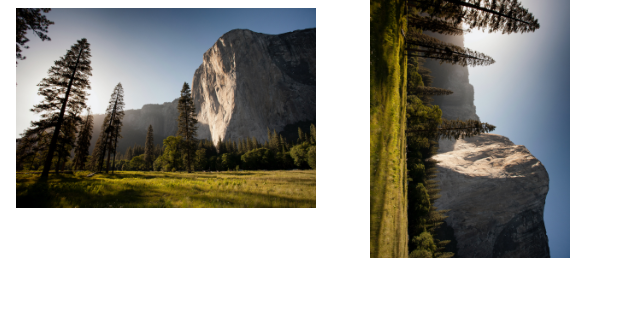First, we access the DOM element object with the document.getElementById() method.

Then, we use the `style.transform` property of the object to set the transform CSS property of the element from JavaScript.

We can specify any angle between `1` and `359` for the rotation:

``````// Access DOM element object
const rotated = document.getElementById('rotated');
// Rotate element by 180 degrees clockwise
rotated.style.transform = 'rotate(180deg)';
``````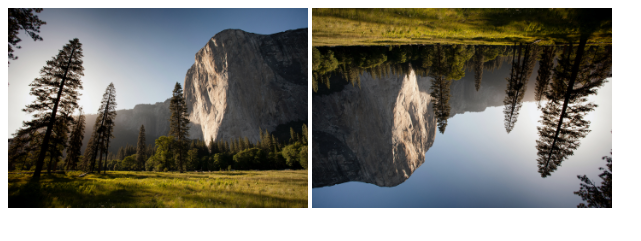### Rotate Image Counter-Clockwise

We can rotate an image in the counter-clockwise direction by specifying a negative angle.

``````// Access DOM element object
const rotated = document.getElementById('rotated');
// Rotate image by 90 degrees counter-clockwise
rotated.style.transform = 'rotate(-90deg)';
``````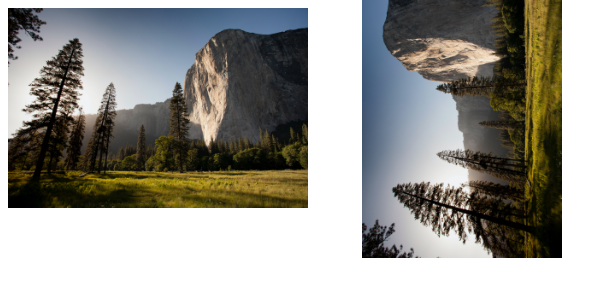We can specify any angle between `-1` and `-359` degrees for counter-clockwise rotation.

``````// Access DOM element object
const rotated = document.getElementById('rotated');
// Rotate image by 135 degrees counter-clockwise
rotated.style.transform = 'rotate(-135deg)';
``````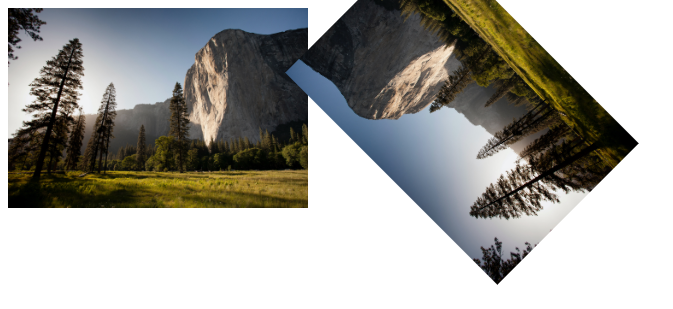### Customize Image Transform Origin

We can use the transform-origin CSS property to set the point that the image will be rotated about. `transform-origin` is `center` by default, which means the element will be rotated about its center point.

In the following example, we rotate the image 90 degrees clockwise, as we did in the first example in this article.

``````// Access DOM element object
const rotated = document.getElementById('rotated');

// Rotate image by 90 degrees clockwise
rotated.style.transform = 'rotate(90deg)';

// Rotate image about top-left corner
rotated.style.transformOrigin = 'top left';
``````

But this time we customize the `transform-origin` property, so the image element ends up at a different position after the rotation.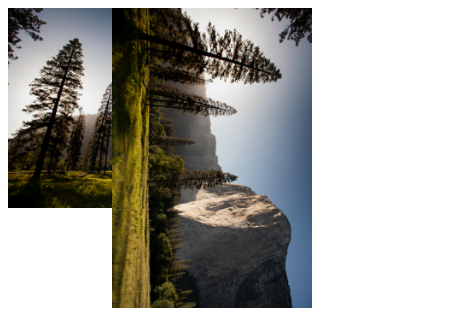### Rotate Image on Button Click

To rotate the image at the click of a button, assign an event handler function to the `onclick` attribute of the `button` element.

For example:

index.html

``````<!DOCTYPE html>
<html>
<title>JavaScript Rotate Image</title>
<body>
<div>
<!-- Assign "rotateImage()" to "onclick" attribute -->
<button id="rotate" onclick="rotateImage()">Rotate image</button>
<!-- The image element to be rotated -->
<img id="rotated" src="my-image.jpg" width="300" />
</div> <script src="index.js"></script>
</body>
</html>
``````

Here the `rotateImage()` function serves as the `click` event handler. It contains the logic for rotating the image and will be called when the button is clicked.

index.js

``````// Access DOM element object
const rotated = document.getElementById('rotated');
function rotateImage() {
// Rotate image by 90 degrees clockwise
rotated.style.transform = 'rotate(90deg)';
}
``````

Now when the button is clicked, the image will be rotated 90 degrees in the clockwise direction.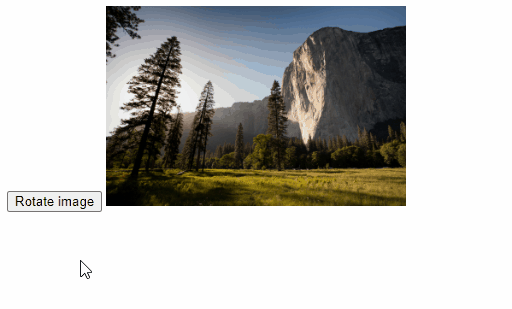### Rotate Image Incrementally

We can incrementally rotate the image on button click by storing the angle of rotation in a variable and using this variable to get and update the current angle. Many image editing apps allow you to rotate images in 90-degree angle increments.

index.js

``````// Access DOM element object
const rotated = document.getElementById('rotated');
// Variable to hold the current angle of rotation
let rotation = 0;
// How much to rotate the image at a time
const angle = 90;
function rotateImage() {
// Ensure angle range of 0 to 359 with "%" operator,
// e.g., 450 -> 90, 360 -> 0, 540 -> 180, etc.
rotation = (rotation + angle) % 360;
rotated.style.transform = `rotate(\${rotation}deg)`;
}
``````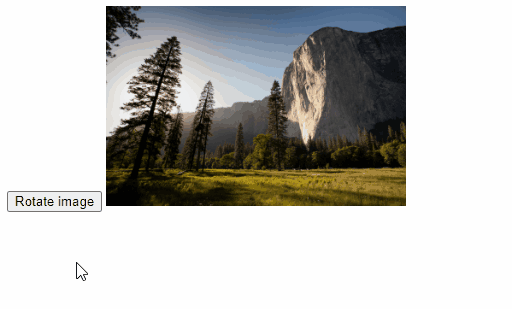That's it for this topic. Thank you for reading.